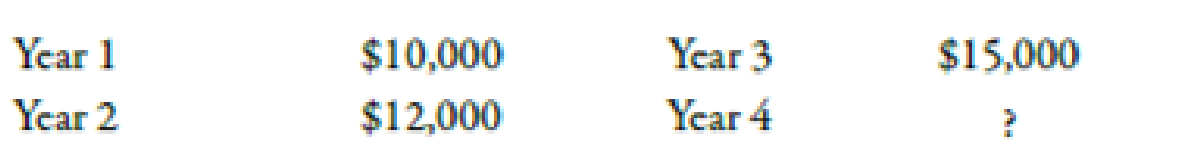Chapter 12, Problem 39E### Managerial Accounting: The Corners...

7th Edition
Maryanne M. Mowen + 2 others
ISBN: 9781337115773

#### Solutions

Chapter
Section### Managerial Accounting: The Corners...

7th Edition
Maryanne M. Mowen + 2 others
ISBN: 9781337115773
Textbook Problem
6 views

# Solving for UnknownsEach of the following scenarios is independent. Assume that all cash flows are after-tax cash flows. a. Thomas Company is investing $120,000 in a project that will yield a uniform series of cash inflows over the next 4 years. b. Video Repair has decided to invest in some new electronic equipment. The equipment will have a 3-year life and will produce a uniform series of cash savings. The NPV of the equipment is$1,750, using a discount rate of 8%. The IRR is 12%. c. A new lathe costing $60,096 will produce savings of$ 12,000 per year. d. The NPV of a project is \$3,927. The project has a life of 4 years and produces the following cash flows:The cost of the project is two times the cash flow produced in Year 4. The discount rate is 10%.Required: 1. If the internal rate of return is 14% for Thomas Company, how much cash inflow per year can be expected? 2. Determine the investment and the amount of cash savings realized each year for Video Repair. 3. For Scenario c, how many years must the lathe last if an IRR of 18% is realized? 4. For Scenario d, find the cost of the project and the cash flow for Year 4.

1.

To determine

Find out the cash flow expected per year if IRR is 14% for TC.

Explanation

Internal Rate of Return:

An interest rate at which the present value of an investment’s cash inflows is equal to the present value cost of the investment is known as internal rate of return. The value of NPV is zero in case of an internal rate of return.

Use the following formula to calculate annual cash flow from the investment:

Annualcashflow(CF)=Investment(I)Disco

2.

To determine

Find out the investment and amount of cash savings realized each year for VR.

3.

To determine

Find out the useful life of lathe if IRR is 18%.

4.

To determine

Calculate the cost of the project and the cash flow for year 4.

### Still sussing out bartleby?

Check out a sample textbook solution.

See a sample solution

#### The Solution to Your Study Problems

Bartleby provides explanations to thousands of textbook problems written by our experts, many with advanced degrees!

Get Started

#### Find more solutions based on key concepts